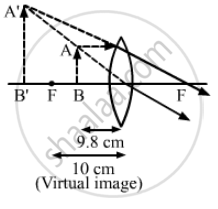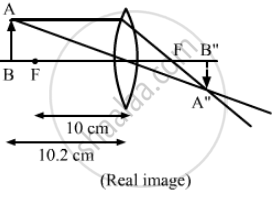Advertisement Remove all ads

# A Convex Lens Has a Focal Length of 10 Cm. Find the Location and Nature of the Image If a Point Object is Placed on the Principal Axis at a Distance of (A) 9.8 Cm, - Physics

Sum

A convex lens has a focal length of 10 cm. Find the location and nature of the image if a point object is placed on the principal axis at a distance of (a) 9.8 cm, (b) 10.2 cm from the lens.

Advertisement Remove all ads

#### Solution

Given:
Focal length (f) of the convex lens = 10 cm

(a) As per the question, the object distance (u) is 9.8 cm.
The lens equation is given by:
$\frac{1}{v} - \frac{1}{u} = \frac{1}{f}$
$\frac{1}{v} = \frac{1}{f} + \frac{1}{u} = \frac{1}{10} - \frac{1}{9 . 8}$
= $\frac{1}{v} = \frac{9 . 8 - 10}{98} = \frac{- 0 . 2}{98}$
= v = − 98 × 5
= − 490 cm (Same side of the object)

v = 490 cm (Virtual and on on the side of object)
Magnification of the image
= v/u

$= \frac{- 490}{- 9 . 8}$

$= 50$
Therefore, the image is erect and virtual.(b) Object distance, u = 10.2 cm
The lens equation is given by:
$\frac{1}{v} - \frac{1}{u} = \frac{1}{f}$
= $\frac{1}{v} = \frac{1}{10} - \frac{1}{10 . 2}$
$= \frac{10 . 2 - 10}{102} = \frac{0 . 2}{102}$
= v = 102 × 5 = 510 cm (Real and on the opposite side of the object)
Magnification of the image $= \frac{v}{u}$

$= \frac{510}{- 9 . 8}$

$= - 52 . 04$
Therefore, the image is real and inverted.Concept: Refraction at Spherical Surfaces and by Lenses - Refraction at Spherical Surfaces
Is there an error in this question or solution?
Advertisement Remove all ads

#### APPEARS IN

HC Verma Class 11, 12 Concepts of Physics 1
Chapter 18 Geometrical Optics
Q 52 | Page 415
Advertisement Remove all ads

#### Video TutorialsVIEW ALL 

Advertisement Remove all ads
Share
Notifications

View all notifications

Forgot password?